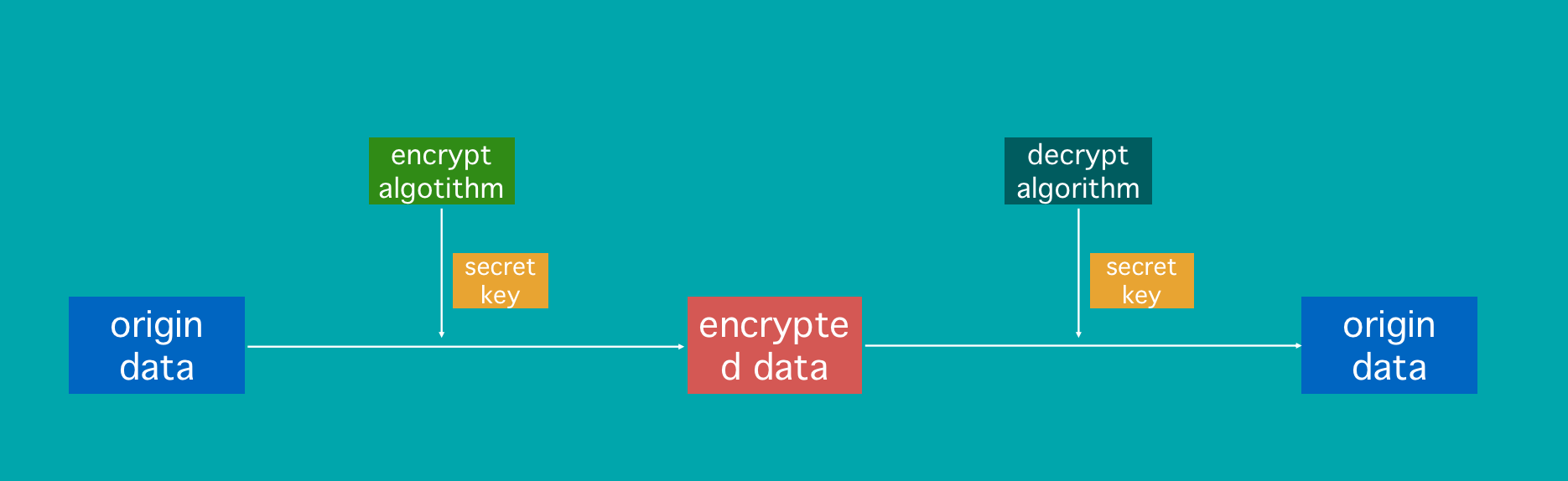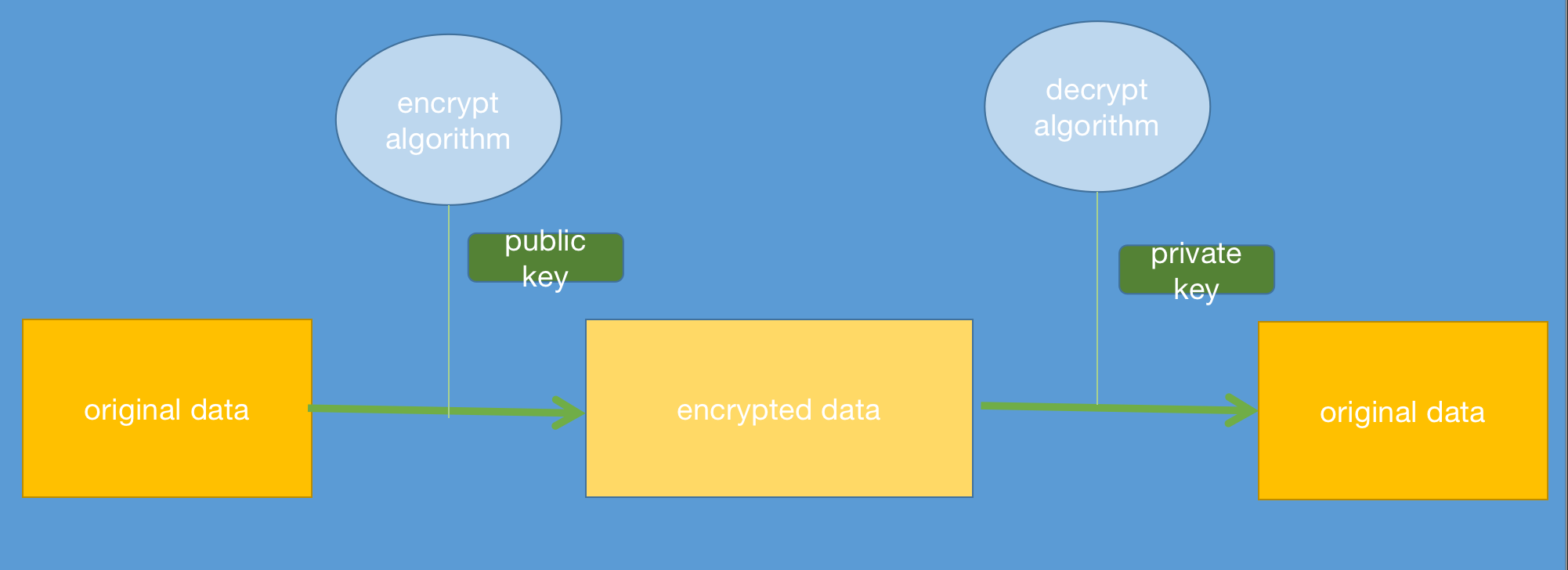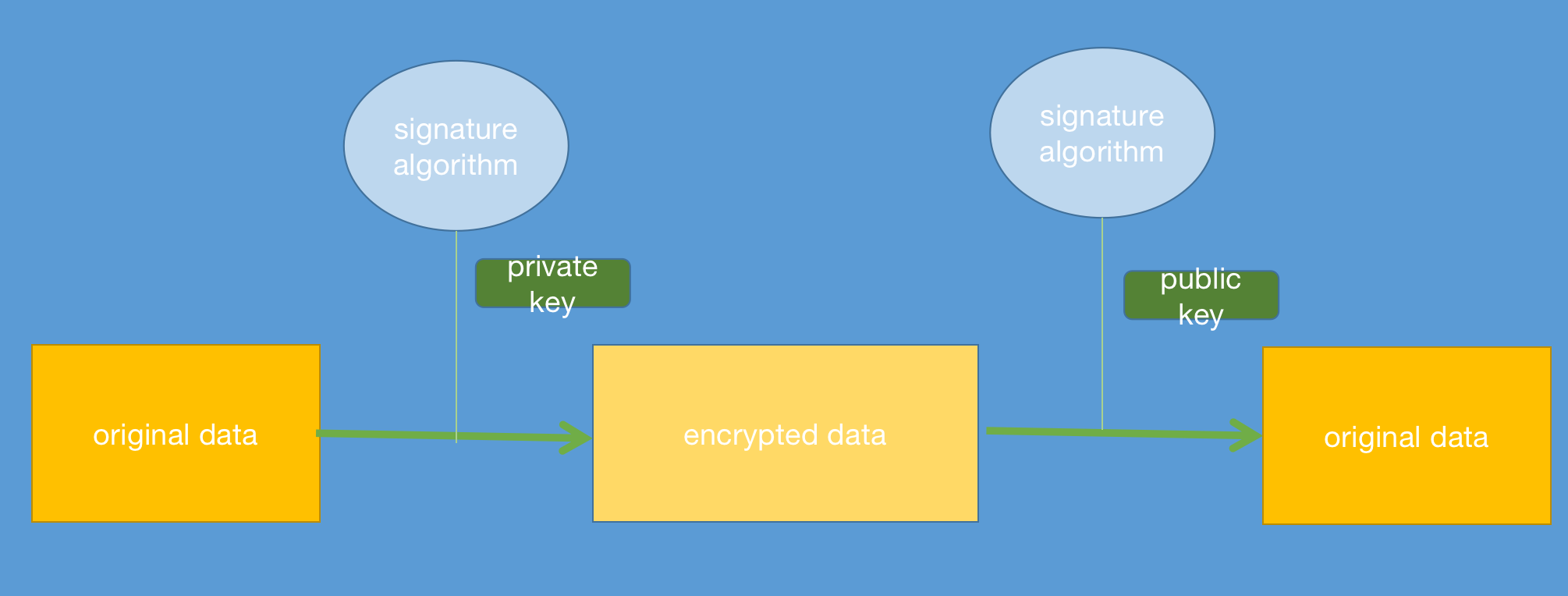# Encryption

There are two useful encrypt algorithms, symmetry encryption and asymmetry encryption.

## Symmetric EncryptionSymmetry encryption use same secret key to encrypt data and decrypt data.

Examples: AES, DES

Disadvantage: The secret problem of symmetry of secret key, it is unsafe to transfer secret key on a unsafe network.

## Asymmetric Encryption

### Base Encrypt and decryptExamples: RSA,AES

Base asymmetry encrypt include public key encrypt and private decrypt.

THis is a base asymmetry encryption, when we use it in network, there is the process:

1. A send its public key to B
2. B send data encrypted by public key
3. A received data and decrypt data with private key, so A got original data

But in this way, we could not avoid man-in-middle attach, if C obtain public key send by A, C can make fake data and send it to A.

### Signature Algorithm

From previous session, we know base asymmetric can not avoid man-in-middle attach, so asymmetric algorithm also have a way to authentication.

We can regard signature algorithm as an reverse of base encrypt algorithm, actually, signature algorithm is a kind of asymmetric algorithm.If we both use base asymmetric and signature algorithm, we can avoid man-in-middle attach in this process:

1. A and B both send other its public key, A got B’s public key [public key B], B got A’s public key [public key A].
2. A send B data(encrypt by [public key B], and add signature data signing by [private key A] to the end of data )
3. B received data from A, and use [private key B] to decrypt encrypted data, and use [public key A] to authentication signature data, if decrypted data is equal with signature data, B can confirm that this data is sent by A, man in middle can not make fake data.

# Encoding

A famous Encoding function is Base64, we use it to convert data to String, so it can display in text, Base64 is not a encrypt algorithm.

# Hash

Hash algorithm includes SHA256, MD5 etc. Hash used in many situation, in java, a base function HashCode, is one of Hash’s uses, it can be regard as a summary or outline of data, and when we use encrypt algorithm, we can use Hash to obtain a better performance.